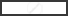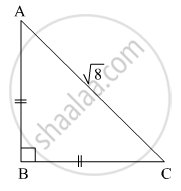Share

# For Finding Ab and Bc with the Help of Information Given in the Figure, Complete Following Activity. Ab = Bc .......... - Geometry

ConceptPythagoras Theorem

#### Question

For finding AB and BC with the help of information given in the figure, complete following activity.

AB = BC ..........$\therefore \angle BAC =$$\therefore AB = BC =$$\times AC$

$=$$\times \sqrt{8}$

$=$$\times 2\sqrt{2}$

=#### Solution

In ∆ABC,
∠B = 90, AC =$\sqrt{8}$ AB = BC, ∴ ∠A = ∠C = 45

By 45∘ − 45 − 90 theorem,

$AB = BC = \frac{1}{\sqrt{2}} \times AC$
$= \frac{1}{\sqrt{2}} \times \sqrt{8}$
$= \frac{1}{\sqrt{2}} \times 2\sqrt{2}$
$= 2$

Hence, AB = 2 and BC = 2.
Hence, the completed activity is

AB = BC .......... Given

$\therefore \angle BAC = {45}^o$
$\therefore AB = BC = \frac{1}{\sqrt{2}} \times AC$
$= \frac{1}{\sqrt{2}} \times \sqrt{8}$
$= \frac{1}{\sqrt{2}} \times 2\sqrt{2}$
$= 2$

Is there an error in this question or solution?

#### Video TutorialsVIEW ALL 

Solution For Finding Ab and Bc with the Help of Information Given in the Figure, Complete Following Activity. Ab = Bc .......... Concept: Pythagoras Theorem.
S